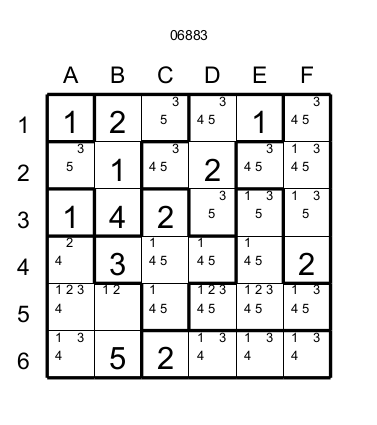Rules of Ripple Effect

# Ripple EffectThe Ripple Effect puzzle (also named "Hakyuu", "Hakyuu Kouka", "Hakyukoka", "Seismic) is similar to Suguru puzzle. There rules are nearly the same,:each puzzle is composed of different regions (delimited by bold lines) of different sizes. Each region of size N must contain numbers from 1 to N. The Ripple Effect differs from the Suguru by:

1) A number N in a cell must not have that the same number in the N neighbouring cells (horizontally or vertically).

2) There is no diagonal constraint. A number N in a cell can touch the same number in one of the four cells in diagonal.

The figure 1 illustrates these rules The cell B4 (yellow) has 3 so the next three cells (green) in any direction (north,south,west and east) from B4 cannot have 3. But the four cells at each corner A3,C3,A5 and C5 can have 3.Figure 1

Another example  (see figure 2)  the cell F2 has 5 then the next five cells (green cells) from F2 cannot have a 5.

Basically, there are 5 kind of technics to resolve a Ripple Effect.

The first two are used in many other puzzles like Suguru, Sudoku, etc... So you should be familiar with these technics.

### 1)  If a cell has a only one candidate then this canidate must be the number in the cell.

The most obvious example is given by previous figure,  the cells D6 and F6 are each one in a region of size 1. So they can have only the number 1. Then D6 and F6  must have 1 (Figure 3).

## For example (Figure 4) the cell  C2 is inside the region having the cells: B1,C2,C3,D3 and D4. This cell C2 has the candidate 4 and this candidate is not present elsewhere in the region ( C2 and D3)  then the cell D2 must have 4.

The following technic is peculiar to the Ripple Effect puzzle.

### 3) If in a region many cells have the same candidate and the cells having this candidate are on a row or a column then it is possible to eliminate this canidate on other cells in the same row or column. Depending of the range of action of the candidate.

For example after resolving this puzzle (Figure 5) with the first two technics we reach this situation (Figure 6).

You can see that cells E3 and F3 are in the same region and that they are in the same row (the third row) and they have candidate 1 and 3 that are not present in the other cell of this region (all the conditions are met to apply the technic 3). These two cells could contain either 1 or 3. If E3 has 1 then D3 cannot have 1 but if F3 has 1 then D3 is not affected by the 1 in F3. But a 3 in any cell E3 or F3 will affect D3. So we can eliminate the candidate 3 from D3 and D3 has only 1. In any case when you face a situation like that take the worst case scenario to eliminate candidate. Suppose the cell B3 and C3 are empty and have the candidate 3 among other candidates. Then the cell E3 affects D3,C3 and B3 and F3 affects D3 and C3 so you could only eliminate the candidate 3 in D3 and C3.

### 4) If in a region only two cells have the same candiate then you could eliminate this candidate on the neighbouring cells that is common of the two ranges of action of the two cells.

For exemple after solving the following Ripple effect (figure 7) with the first three technics.

We reach the following situation (Figure 8)Figure 8

The cells D3 and E4 are the only two cells in this region to have  a same candidate: 2. Then we can eliminate the candidate 2 for all the other cells under the range of action of D3 and E4. The only cell which is affected by D3 and E4 by the candidate 3 is D4 This mean that if D3 has 2 then the candidate 2 in D4 will be eradicated and the same thing happens if E4 has 2.

And finaly the last resort technic

### 5) If a cell contains a number and this number generates an invalid situation (for example a candidate is tolally eliminated in a region) then  this cell cannot have this candidate and we can eliminate gleefully this candidate from this cell.

For exemple after solving the following Ripple effect (figure 9) with the first four technics.

We reach the following situation (Figure 10)Figure 10

The cell C2 has candidates 3,4 and 5. If C2 has 3, it will eliminate the candidate 3 in cells A2 and C1 so this region will be invalid. The same thing happens if C2 has 5. Then we could remove the candidates 3 and 5 from C2 and finally only the candidate 4 remains in C2. In this example the effect is easily measurable. But in some case (the most difficult puzzles) the effect could result from a chain of forced moves

I have graded my puzzles by the number of technics used (1 star for technic 1, 2 for technic 2, etc..)

To conclude if you like Suguru you will like the Ripple Effect. The Ripple Effect is stimulating to solve.

Enjoy !!